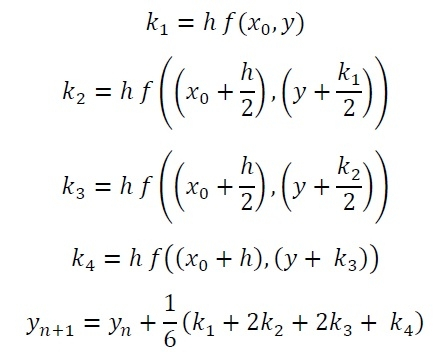# Runge-Kutta 4th order rule for differential equation

Runge Kutta method is used for solving ordinary differential equations (ODE). It uses dy/dx function for x and y, and also need the initial value of y, i.e. y(0). It finds the approximate value of y for given x. For solving ODE, we have to follow these formulas:Here h is the height of the interval.

Note: From these formulas, we can use first two k1 and k2 find the Runge-Kutta 2nd Order solution for ODE.

## Input and Output

Input:
The x0 and f(x0): 0 and 0
the value of x = 0.4
the value of h = 0.1
Output:
Answer of differential equation: 0.0213594

## Algorithm

rungeKutta(x0, y0, x, h)

Input − Initial x and y value, the targeted x value, and the height of interval h.

Output − The value of y for value x.

Begin
iteration := (x – x0)/h
y = y0
for i := 1 to iteration, do
k1 := h*f(x0, y)
k2 := h*f((x0 + h/2), (y + k1/2))
k3 := h*f((x0 + h/2), (y + k2/2))
k4 := h*f((x0 + h), (y + k3))
y := y + (1/6)*(k1 + 2k2 + 2k3 + k4)
x0 := x0 + h
done
return y
End

## Example

#include <iostream>
using namespace std;

double diffOfy(double x, double y) {
return ((x*x)+(y*y)); //function x^2 + y^2
}

double rk4thOrder(double x0, double y0, double x, double h) {
int iteration = int((x - x0)/h);    //calculate number of iterations
double k1, k2, k3, k4;
double y = y0;    //initially y is f(x0)

for(int i = 1; i<=iteration; i++) {
k1 = h*diffOfy(x0, y);
k2 = h*diffOfy((x0+h/2), (y+k1/2));
k3 = h*diffOfy((x0+h/2), (y+k2/2));
k4 = h*diffOfy((x0+h), (y+k3));

y += double((1.0/6.0)*(k1+2*k2+2*k3+k4));    //update y using del y
x0 += h;    //update x0 by h
}
return y;    //f(x) value
}

int main() {
double x0, y0, x, h;
cout << "Enter x0 and f(x0): "; cin >> x0 >> y0;
cout << "Enter x: "; cin >> x;
cout << "Enter h: "; cin >> h;
cout << "Answer of differential equation: " << rk4thOrder(x0, y0, x, h);
}

## Output

Enter x0 and f(x0): 0 0
Enter x: 0.4
Enter h: 0.1
Answer of differential equation: 0.0213594